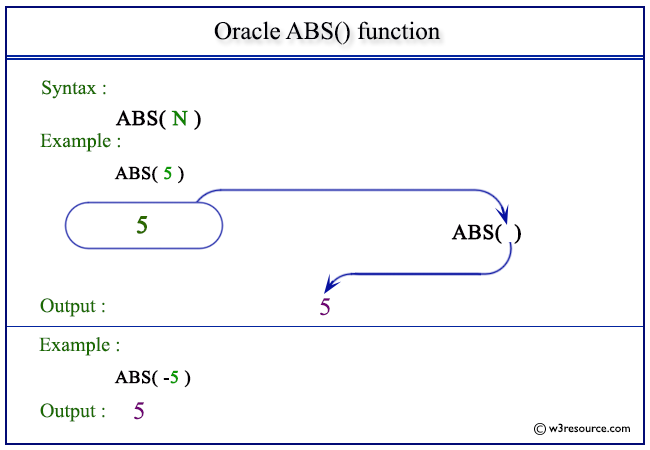# Oracle ABS() function

## Description

The ABS() function is used to calculate the absolute value of an expression.

The function takes any numeric or nonnumeric data type (can be implicitly converted to a numeric data type) as an argument.
The function returns the same data type as the numeric data type of the argument.

Syntax:

` ABS(N); `

Parameters:

Name Description
N A number whose absolute value is to be retrieved.

Pictorial Presentation of ABS() functionExample: ABS() function using positive value

The example below shows that, the oracle statement will return the absolute value of a positive number specified in the argument.

``````SELECT ABS(5) FROM dual;
```
```

Here is the result.

```    ABS(5)
----------
5
```

Example: ABS() function using negative value

The example below shows that, the oracle statement will return the absolute value of a negative number defined in the argument.

```SELECT ABS(-5) FROM dual;
```

Here is the output.

```    ABS(5)
----------
5
```

Previous: Oracle Numeric Functions Introduction
Next: ACOS

﻿